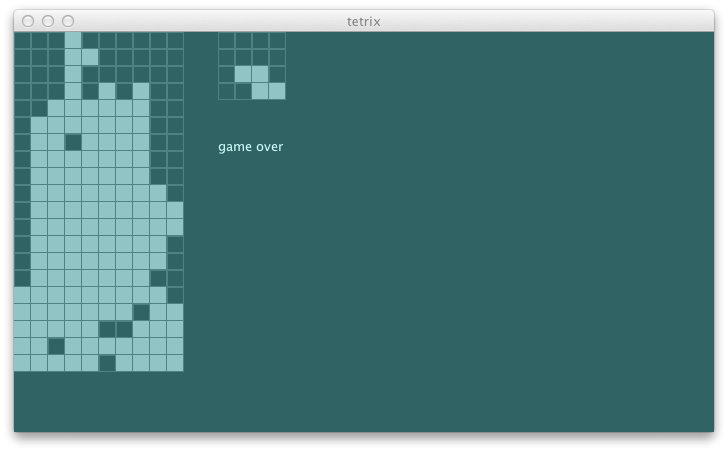### pruning the search tree

Pruning allows us to ignore portion of the search tree that make no difference to the final choice.

This concept applies here because without control the search tree grows exponentially.

1. We can reduce the branching factor from five to tree by omitting `Drop` and `Tick`. We already pretend that the current pieces gets dropped. All we have to do is to evaluate `s0` with `Drop`.
2. Next, we can eliminate `RotateCW` by pre-branching for all four orientations of the current piece. In most cases `RotateCW :: MoveLeft :: RotateCW :: Drop :: Nil` and `RotateCW :: RotateCW :: MoveLeft :: Drop :: Nil` brings us to the same state.
3. We can get rid of `MoveLeft` by moving the current piece as left as it can upfront.

Potentially a piece can have four orientations and nine x-position. Thus, exponential tree search tree can now be approximated by a constant size 36.

First, we can list out the possible orientations based on the `PieceKind`:

``````  private[this] def orientation(kind: PieceKind): Int = {
case IKind => 2
case JKind => 4
case LKind => 4
case OKind => 1
case SKind => 2
case TKind => 4
case ZKind => 2
}
``````

Next, given a state calculate how many times can the agent hit left or right using the REPL.

``````scala> val s = newState(Nil, (10, 20), TKind :: TKind :: Nil)
s: com.eed3si9n.tetrix.GameState = GameState(List(
Block((4,18),TKind), Block((5,18),TKind), Block((6,18),TKind), Block((5,19),TKind)),
(10,20),Piece((5.0,18.0),TKind,List((-1.0,0.0), (0.0,0.0), (1.0,0.0), (0.0,1.0))),
Piece((2.0,1.0),TKind,List((-1.0,0.0), (0.0,0.0), (1.0,0.0), (0.0,1.0))),List(),ActiveStatus,0)

scala> import scala.annotation.tailrec
import scala.annotation.tailrec

scala> @tailrec def leftLimit(n: Int, s: GameState): Int = {
val next = moveLeft(s)
if (next.currentPiece.pos == s.currentPiece.pos) n
else leftLimit(n + 1, next)
}
leftLimit: (n: Int, s: com.eed3si9n.tetrix.GameState)Int

scala> leftLimit(0, s)
res1: Int = 4
``````

Make the same one for right, and we have `sideLimit` method:

``````  private[this] def sideLimit(s0: GameState): (Int, Int) = {
@tailrec def leftLimit(n: Int, s: GameState): Int = {
val next = moveLeft(s)
if (next.currentPiece.pos == s.currentPiece.pos) n
else leftLimit(n + 1, next)
}
@tailrec def rightLimit(n: Int, s: GameState): Int = {
val next = moveRight(s)
if (next.currentPiece.pos == s.currentPiece.pos) n
else rightLimit(n + 1, next)
}
(leftLimit(0, s0), rightLimit(0, s0))
}
``````

These should be enough to build `actionSeqs`:

``````                                                                              s2"""
ActionSeqs function should
list out potential action sequences                                       \$actionSeqs1
"""
...
def actionSeqs1 = {
val s = newState(Nil, (10, 20), TKind :: TKind :: Nil)
val seqs = agent.actionSeqs(s)
seqs.size must_== 32
}
``````

Stub it out:

``````  def actionSeqs(s0: GameState): Seq[Seq[StageMessage]] = Nil
``````

The test fails as expected:

``````[info] ActionSeqs function should
[error] x list out potential action sequences
[error]    '0' is not equal to '32' (AgentSpec.scala:15)
``````

Here’s the implementation:

``````  def actionSeqs(s0: GameState): Seq[Seq[StageMessage]] = {
val rotationSeqs: Seq[Seq[StageMessage]] =
(0 to orientation(s0.currentPiece.kind) - 1).toSeq map { x =>
}
val translationSeqs: Seq[Seq[StageMessage]] =
sideLimit(s0) match {
case (l, r) =>
((1 to l).toSeq map { x =>
}) ++
Seq(Nil) ++
((1 to r).toSeq map { x =>
})
}
for {
r <- rotationSeqs
t <- translationSeqs
} yield r ++ t
}
``````

We can see the output using REPL:

``````scala> val s = newState(Nil, (10, 20), TKind :: TKind :: Nil)
s: com.eed3si9n.tetrix.GameState = GameState(List(Block((4,18),TKind), Block((5,18),TKind),
Block((6,18),TKind), Block((5,19),TKind)),(10,20),
Piece((5.0,18.0),TKind,List((-1.0,0.0), (0.0,0.0), (1.0,0.0), (0.0,1.0))),
Piece((2.0,1.0),TKind,List((-1.0,0.0), (0.0,0.0), (1.0,0.0), (0.0,1.0))),List(),ActiveStatus,0)

scala> val agent = new Agent
agent: com.eed3si9n.tetrix.Agent = com.eed3si9n.tetrix.Agent@649f7367

scala> agent.actionSeqs(s)
res0: Seq[Seq[com.eed3si9n.tetrix.StageMessage]] = Vector(List(MoveLeft),
List(MoveLeft, MoveLeft), List(MoveLeft, MoveLeft, MoveLeft),
List(MoveLeft, MoveLeft, MoveLeft, MoveLeft), List(), List(MoveRight),
List(MoveRight, MoveRight), List(MoveRight, MoveRight, MoveRight),
List(RotateCW, MoveLeft), List(RotateCW, MoveLeft, MoveLeft),
List(RotateCW, MoveLeft, MoveLeft, MoveLeft),
List(RotateCW, MoveLeft, MoveLeft, MoveLeft, MoveLeft), List(RotateCW),
List(RotateCW, MoveRight), List(RotateCW, MoveRight, MoveRight),
List(RotateCW, MoveRight, MoveRight, MoveRight), List(RotateCW, RotateCW, MoveLeft),
List(RotateCW, RotateCW, MoveLeft, MoveLeft),
List(RotateCW, RotateCW, MoveLeft, MoveLeft, MoveLeft),
List(RotateCW, RotateCW, MoveLeft, MoveLeft, MoveLeft, MoveLeft),
List(RotateCW, RotateCW),...
``````

Note one of the action sequences is `List()`, which evaluates the current state. All tests pass too:

``````[info] ActionSeqs function should
[info] + list out potential action sequences
``````

We can now rewrite `bestMove` using `actionSeqs`:

``````  def bestMove(s0: GameState): StageMessage = {
var retval: Seq[StageMessage] = Nil
var current: Double = minUtility
actionSeqs(s0) foreach { seq =>
val ms = seq ++ Seq(Drop)
val u = utility(Function.chain(ms map {toTrans})(s0))
if (u > current) {
current = u
retval = seq
} // if
}
println("selected " + retval + " " + current.toString)
}
``````

Now let’s add more spec. How about having a single gap open at `(0, 8)` such that it requires several rotations and a bunch of `MoveRight`s? This is something our agent would have not solved before.

``````                                                                              s2"""
Solver should
pick MoveLeft for s1                                                      \$solver1
pick Drop for s3                                                          \$solver2
pick RotateCW for s5                                                      \$solver3
"""
...
def s5 = newState(Seq(
(0, 0), (1, 0), (2, 0), (3, 0), (4, 0), (5, 0), (6, 0),
(7, 0), (9, 0))
map { Block(_, TKind) }, (10, 20), ttt)
def solver3 =
agent.bestMove(s5) must_== RotateCW
``````

All green. Now let’s run the swing UI to see how it looks:``````[info] selected List(RotateCW, MoveLeft, MoveLeft, MoveLeft, MoveLeft) 1.4316304877998318
[info] selected List(MoveLeft, MoveLeft, MoveLeft, MoveLeft) 1.4316304877998318
[info] selected List(MoveLeft, MoveLeft, MoveLeft) 1.4316304877998318
[info] selected List(MoveLeft, MoveLeft) 1.4316304877998318
[info] selected List() 1.4108824377664941
``````

It is rather short-sighted in its moves, but I am starting to see the glimpse of rationality. We’ll pick it up from here tomorrow.

``````\$ git fetch origin
\$ git co day8v2 -b try/day8
\$ sbt swing/run
``````